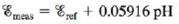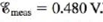# The measurement of pH using a glass electrode obeys the Nernst equation. The typical response of a pH meter at 25 00°C is given by the equation where contains the potential of the reference electrode and all other potentials that arise in the cell that are not related to the hydrogen ion concentration. Assume that = 0.250 V and that a. What is the uncertainty in the values of pH and [H + ] if the uncertainty in the measured potential is ±1 m V (± 0.001 V)? b. To what precision must the potential be measured for the uncertainty in pH to be ± 0.02 pH unit?### Chemistry: An Atoms First Approach

2nd Edition
Steven S. Zumdahl + 1 other
Publisher: Cengage Learning
ISBN: 9781305079243

#### Solutions

Chapter
Section### Chemistry: An Atoms First Approach

2nd Edition
Steven S. Zumdahl + 1 other
Publisher: Cengage Learning
ISBN: 9781305079243
Chapter 17, Problem 147CP
Textbook Problem
21 views

## The measurement of pH using a glass electrode obeys the Nernst equation. The typical response of a pH meter at 25 00°C is given by the equationwherecontains the potential of the reference electrode and all other potentials that arise in the cell that are not related to the hydrogen ion concentration. Assume that= 0.250 V and thata. What is the uncertainty in the values of pH and [H+] if the uncertainty in the measured potential is ±1 m V (± 0.001 V)?b. To what precision must the potential be measured for the uncertainty in pH to be ± 0.02 pH unit?

(a)

Interpretation Introduction

Interpretation: The uncertainty in the values of pH and [H+] is to be calculated. The potential for the uncertainty in pH is to be measured.

Concept introduction: Cell potential is defined as the measure of energy per unit charge available from the redox reaction to carry out the reaction. The uncertainty is the measured values up to only significant figures or numbers.

To determine: The uncertainty in the values of pH and [H+] .

### Explanation of Solution

Explanation

Given

The equation that states a typical response of a pH meter at 25°C .

Emeas=Eref+0.05916 pH

Emeas=0.480 V

Eref=0.250 V

The uncertainty in the measured potential is ±1 mV(±0.001 V)

The first step is to calculate the pH by substituting the values of Emeas and Eref in the given equation above as follows,

Emeas=Eref+0.05916 pH0.480 V=0.250 V+0.05916 pHpH=0.480 V0.250 V0.05916=3.888

The uncertainty in the measured potential is ±1 mV(±0.001 V) .

Thus, the measured potential is a maximum of 0.481 V or a minimum of 0.479 V . Using these values, the maximum and minimum pH is calculated by using the given expression,

Emeas=Eref+0.05916 pHpH=EmeasEref0.05916

Substitute the values of Emeas and Eref in the above expression to calculate the pHmax .

pHmax=EmeasEref0.05916pHmax=0.481 V0.250 V0.05916=3.905

Similarly, minimum pH is calculated by substituting the values of Emeas and Eref in the above expression.

pHmin=EmeasEref0.05916pHmin=0.479 V0.250 V0.05916=3.871

Hence, the uncertainty in pH is calculated as follows,

Uncertainty=±(pHmaxpHmin)=±(3.9053.888)=±0

(b)

Interpretation Introduction

Interpretation: The uncertainty in the values of pH and [H+] is to be calculated. The potential for the uncertainty in pH is to be measured.

Concept introduction: Cell potential is defined as the measure of energy per unit charge available from the redox reaction to carry out the reaction. The uncertainty is the measured values up to only significant figures or numbers.

To determine: The potential for the uncertainty in pH .

### Still sussing out bartleby?

Check out a sample textbook solution.

See a sample solution

#### The Solution to Your Study Problems

Bartleby provides explanations to thousands of textbook problems written by our experts, many with advanced degrees!

Get Started

Find more solutions based on key concepts
What is the mass of a 43.8-mL sample of gasoline, which has a density of 0.70 g/cm3?

General Chemistry - Standalone book (MindTap Course List)

Blood LDL values of people eating typical, meat-rich Western diets are generally higher than LDL values of vege...

Nutrition: Concepts and Controversies - Standalone book (MindTap Course List)

22. Name each compound:

Chemistry In Focus

What are common epigenetic changes?

Introduction to General, Organic and Biochemistry

Two blocks connected by a rope of negligible mass are being dragged by a horizontal force (Fig. P5.65). Suppose...

Physics for Scientists and Engineers, Technology Update (No access codes included)

What is an extremophile?

Oceanography: An Invitation To Marine Science, Loose-leaf Versin